# seapy.components.beam.SubsystemShear¶

class seapy.components.beam.SubsystemShear(name, system, **properties)[source]

Subsystem for shear waves in a 1D isotropic system.

__init__(name, system, **properties)

Constructor.

Parameters: name (string) – Identifier component (SeaPy.components.Component) – Component

Methods

 __init__(name, system, **properties) Constructor. addExcitation(name, model, **properties) Add excitation to subsystem. disable([couplings]) Disable this subsystem. enable([couplings]) Enable this subsystem. info([attributes]) Return dataframe. plot(quantity[, yscale]) Plot quantity.

Attributes

 SORT str(object=’‘) -> str average_frequency_spacing Average frequency spacing for bending waves. classname Name of class of the object. component conductance Conductance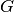. conductance_point_average Average point conductance of a structural component. damping_term The damping term is the ratio of the modal half-power bandwidth to the average modal frequency spacing. dlf Damping loss factor of subsystem. enabled Switch indicating whether the object is enabled. energy Total energy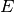in subsystem. frequency Frequency. impedance Impedance included Indicates whether the object is included in the analysis. linked_couplings_from linked_couplings_to linked_excitations mobility Mobility Y modal_density Modal density. modal_energy Class capable of containing spectral values. modal_overlap_factor Modal overlap factor. name power_input Total input power due to excitations. resistance Resistance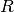, the real part of the impedance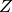. resistance_point_average Average point resistance. soundspeed_group Group velocity for shear wave. soundspeed_phase Phase velocity for shear wave. tlf Total loss factor. velocity Vibrational velocity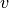. velocity_level Velocity level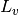. wavenumber Wave number.
average_frequency_spacing[source]

Average frequency spacing for bending waves.

Return type: numpy.ndarray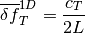See Lyon, eq. 8.1.8

impedance[source]

Impedance

Return type: numpy.ndarray
soundspeed_group[source]

Group velocity for shear wave.

Return type: numpy.ndarray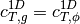soundspeed_phase[source]

Phase velocity for shear wave.

Return type: numpy.ndarray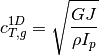#### Previous topic

seapy.components.beam.SubsystemBend

#### Next topic

seapy.components.plate.Component2DPlate